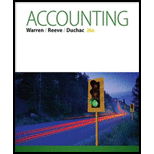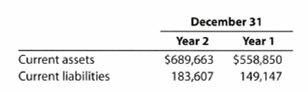Chapter 4, Problem 4.21EX### Accounting (Text Only)

26th Edition
Carl Warren + 2 others
ISBN: 9781285743615

#### Solutions

Chapter
Section### Accounting (Text Only)

26th Edition
Carl Warren + 2 others
ISBN: 9781285743615
Textbook Problem
9 views

# Working capital and current ratioThe following data (in thousands) were taken from recent financial statements of Under Armour, Inc.:a. Compute the working capital and the current ratio as of December 31, Year 2 and Year 1. Round to two decimal places.b. What conclusions concerning the company's ability to meet its financial obligations can you draw from part (a)?

(a)

To determine

Ratio analysis

It is the financial analysis tool for measuring the profitability, liquidity, capability and overall performance of a company.

Following are the two measures of liquidity:

1. 1. Current ratio: Current ratio is used to determine the relationship between current assets and current liabilities. The ideal current ratio is 2:1. The following formula is used to calculate current ratio.
2. 2. Working capital: Total current assets minus total current liabilities are the working capital of a company.

To Compute:  The working capital and current ratio as of December 31 for Year 2 and Year 1.

Explanation
 Details Year 2 ($) Year 1 ($) Current assets 689,663 558,850 Less: Current liabilities (183,607) (149,147) Working capital $506,056$409,703

Table (1)

Calculate the current ratio for Year 2 and Year 1.

Current ratio for year 2=Current assetsCurrent

(b)

To determine

To Explain: The ability of a company to meet its financial obligations.

### Still sussing out bartleby?

Check out a sample textbook solution.

See a sample solution

#### The Solution to Your Study Problems

Bartleby provides explanations to thousands of textbook problems written by our experts, many with advanced degrees!

Get Started

#### Find more solutions based on key concepts